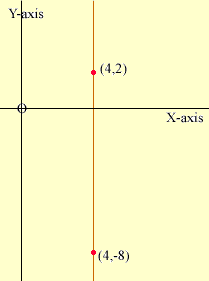Quandaries and Queries Hello,   Herein lies the problem: Write an equation for the line. Given points (4, -8) and (4,2) are on the line. I believe m=undefined.   How do I solve for b when m is undefined, and then plug in the values for m, b, and y?   Thanks! Kim Hi Kim, The first thing to do is to sketch a diagram of the plane with the points on it and the line through the points.What you see imediately, even if you didn't see it from the numbers, is that the line is vertical. But the diagram also shows you a way to describe all the points in the plane that lie on the line. The points on the line are precisely the points in the plane that have first co-ordinate equal to 4. That is they are the point in the plane that satisfy x = 4. This is the equation of the line. It is an algebraic expression that describes exactly what points are on the line. Penny Go to Math Central